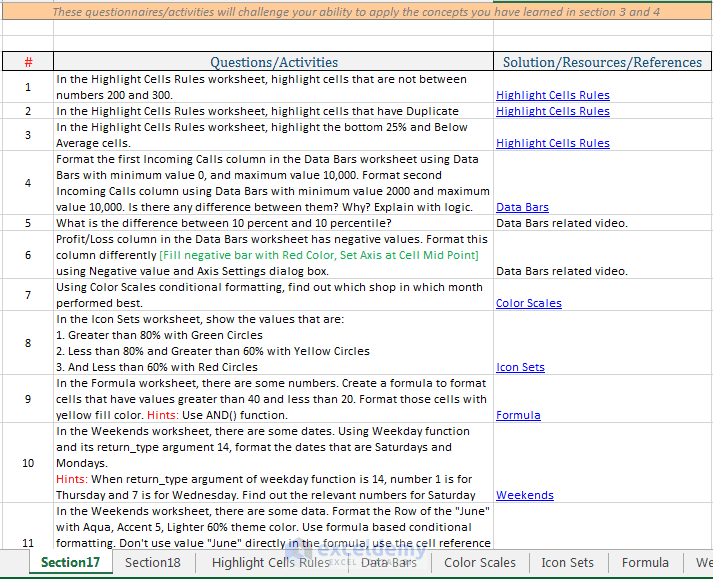# Excel Conditional Formatting Formula

In this tutorial Excel Conditional Formatting Formulas, you will learn how to use Excel formulas to format cells conditionally.

In this tutorial, you have been introduced with the basic conditional formatting.

You might want to look through the other posts related to Excel Conditional Formatting Formula:

## Excel Conditional Formatting Formula

In this video lecture:

• You will learn how to format cells using a formula based conditional formatting.
• You will learn about ISTEXT() Function.
• And you will learn again how to use Absolute Referencing in formulas.## Video Transcript

You can format any cell using conditional formatting rules. We have so far learned how to highlight cells based on the cell content. We have used these Greater than, Less than, Between, Equal to, and other formatting rules. We have also learned how to highlight some top or bottom values. We have used Data bars, Color Scales and Icon Sets to format cells conditionally. In this video tutorial and in the next video, we shall learn how you can format cells using formulas. You see a piece of the data range in this worksheet. The range has some values and has some texts. For example, say, I am telling you to format the cells differently that have texts. You cannot use the techniques that you have learned so far to perform this job. You have to use a formula. I select the first cell within the range, then click on the conditional formatting drop-down, then click on New Rule. New Formatting Rule dialog box appears. In the dialog box, select the option: “Use a Formula to determine which cells to format”. You see there is a field under: “Format values where this formula is true”. You have to put the formula in this field. If it returns True, then the cell will be formatted. So, the formula that you can use in this field must return either true or false.

Let’s clear the concept with an example. I input equal sign; you know an equal sign is a must thing to write a formula. Then I type ISTEXT, open parenthesis, type cell reference A3, closing parenthesis. The ISTEXT function returns True if the argument of the function is a text value. I click on the Format Button, Format Cells dialog box appears, I use Bold Font style, Font color Red. I click OK, you see the preview here. If this function returns true, then the format you set here will be seen in the cell. If the function returns False, then no format will be applied on a cell. I click OK. You see the cell is not formatted. The value of cell A3 is not a text, so this function returns False and the cell is not formatted. Now I copy the Format from this cell and paste the format to other cells in the range. You see the cells that have texts, are highlighted with Boldface and Red color Font. One thing, you have to understand very clearly here: when I copied the formatting from this cell to other cells, the format is copied to other cells using relative references. I created a formula referring cell A3,

so if I copy this cell format, and paste format in the next cell, the formula will now refer to cell B4. With another example, you will get clear the concept. In the next worksheet, you see there are some values in this data range. Say I want to highlight the cells that have value 38. You can do it using the “Equal To” rule in the “Highlight Cells Rules” list. But this time I shall use formula. I select all the data here; you have to make sure that the first cell in the range is selected. In my selection, the first cell is selected. Now conditional formatting drop-down, New rule, New Formatting Rule dialog box appears, select option “Use a Formula to determine which cells to Format”. In the formula field, I type =, A3, equal to C3. Click on the Format button, the Format Cells dialog box appears, Red color font and Bold Face. So if the cell A3 is equal to cell C3, then the cell will be highlighted. You see the cells that have 38 are not formatted. But this cell has 38; this cell has 38 and so on. So what is the problem with this formula? The problem is in cell referencing. For the first cell the formula is A3 equal to C3, but for the next cell the formula is B3 equal to D3, D3 has no value. For the next cell, the formula is A4 equal to C4, C4 has no value. But every time the second cell must be cell C3. OK, let’s manage the formula. Select any cell in the range, conditional formatting, Manage Rules, Edit Rule button, I select the C3 cell and press F4 key in the keyboard. The cell C3 is now turned into absolute cell referencing. I click OK, I click on Apply, and OK. You see the cells that have 38 as value, are highlighted with Red Font Color and Boldface. This is the basis of using a formula to format cells conditionally. In the next video; we shall use more examples to make the concept clearer to you.

How to Highlight Every Other Row in Excel

Excel Conditional Formatting Dates

Excel Conditional Formatting Based on Another Cell

Excel Conditional Formatting Based on Another Cell Text

#### Kawser

Hello! Welcome to my Excel blog! It took me some time to be a fan of Excel. But now I am a die-hard fan of MS Excel. I learn new ways of doing things with Excel and share here. Not only how to guide on Excel, but you will get also topics on Finance, Statistics, Data Analysis, and BI. Stay tuned!

1 Comment